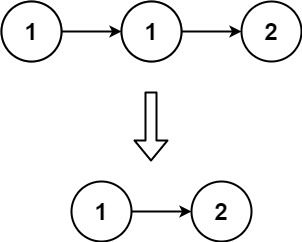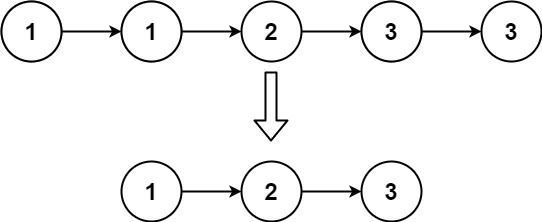# 83. Remove Duplicates from Sorted List

Given the `head` of a sorted linked list, delete all duplicates such that each element appears only once. Return the linked list sorted as well.

Example 1:```Input: head = [1,1,2]
Output: [1,2]
```

Example 2:```Input: head = [1,1,2,3,3]
Output: [1,2,3]
```

Constraints:

• The number of nodes in the list is in the range `[0, 300]`.
• `-100 <= Node.val <= 100`
• The list is guaranteed to be sorted in ascending order.

## The solution to the above question is -

/**
* struct ListNode {
*     int val;
*     ListNode *next;
*     ListNode() : val(0), next(nullptr) {}
*     ListNode(int x) : val(x), next(nullptr) {}
*     ListNode(int x, ListNode *next) : val(x), next(next) {}
* };
*/
class Solution {
public:
int prev=temp->val;
while(temp!=NULL){
if( temp->next!=NULL && temp->next->val==prev){
temp->next=temp->next->next;
}
else{
temp=temp->next;
if(temp!=NULL)
prev=temp->val;
cout<<prev<<" ";
}
}